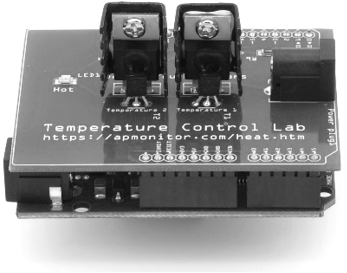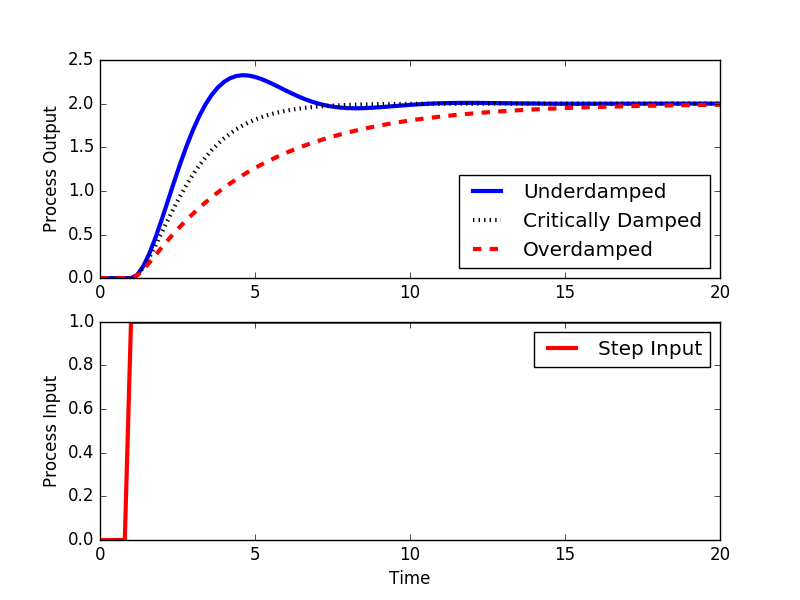## Second Order SystemsA second-order linear system is a common description of many dynamic processes. The response depends on whether it is an overdamped, critically damped, or underdamped second order system.

$$\tau_s^2 \frac{d^2y}{dt^2} + 2 \zeta \tau_s \frac{dy}{dt} + y = K_p \, u\left(t-\theta_p \right)$$

has output y(t) and input u(t) and four unknown parameters. The four parameters are the gain K_p, damping factor \zeta, second order time constant \tau_s, and dead time \theta_p.

#### Laplace Domain, Transfer Function

In the Laplace domain, the second order system is a transfer function:

$$\frac{Y(s)}{U(s)} = \frac{K_p}{\tau_s^2 s^2 + 2 \zeta \tau_s s + 1}e^{-\theta_p s}$$

#### State Space Form

To put the second order equation into state space form, it is split into two first order differential equations.

$$\frac{dx_1}{dt} = x_2$$

$$\tau_s^2 \frac{dx_2}{dt} = -2 \zeta \tau_s x_2 - x_1 + K_p u\left(t-\theta_p\right)$$

State x_1 is the output in state space form.

$$\begin{bmatrix}\dot x_1\\\dot x_2\end{bmatrix} = \begin{bmatrix}0&1\\-\frac{1}{\tau_s^2}&-\frac{2 \zeta}{\tau_s}\end{bmatrix} \begin{bmatrix}x_1\\x_2\end{bmatrix} + \begin{bmatrix}0\\\frac{K_p}{\tau_{s}^2}\end{bmatrix} u\left(t-\theta_p\right)$$

$$y = \begin{bmatrix}1 & 0\end{bmatrix} \begin{bmatrix}x_1\\x_2\end{bmatrix} + \begin{bmatrix}0\end{bmatrix} u$$

#### Process Gain, K_p

The process gain is the change in the output y induced by a unit change in the input u. The process gain is calculated by evaluating the change in y(t) divided by the change in u(t) at steady state initial and final conditions.

$$K_p = \frac{\Delta y}{\Delta u} = \frac{y_{ss_2}-y_{ss_1}}{u_{ss_2}-u_{ss_1}}$$

The process gain affects the magnitude of the response, regardless of the speed of response.

#### Damping Factor

The response of the second order system to a step input in u(t) depends whether the system is overdamped (\zeta>1), critically damped (\zeta=1), or underdamped (0 \le \zeta < 1).##### 1. Overdamped

If the system is overdamped (\zeta>1), the analytic solution to the step response of magnitude M is

$$y(t) = K_p M \left( 1-e^{-\zeta\,t/\tau_s} \left[ \cosh\left( \frac{t}{\tau_s}\sqrt{\zeta^2 - 1} \right) + \frac{\zeta}{\sqrt{\zeta^2-1}} \sinh\left( \frac{t}{\tau_s}\sqrt{\zeta^2 - 1} \right) \right] \right)$$

##### 2. Critically Damped

If the system is critically damped (\zeta=1), the analytic solution to the step response of magnitude M is

$$y(t) = K_p M \left[ 1 - \left( 1+\frac{t}{\tau_s} \right) e^{-t/\tau_s} \right]$$

##### 3. Underdamped (oscillations)

Finally, if the system is underdamped (0\le\zeta<1), the analytic solution to the step response of magnitude M is

$$y(t) = K_p M \left( 1-e^{-\zeta\,t/\tau_s} \left[ \cos\left( \frac{t}{\tau_s}\sqrt{1-\zeta^2} \right) + \frac{\zeta}{\sqrt{1-\zeta^2}} \sin\left( \frac{t}{\tau_s}\sqrt{1-\zeta^2} \right) \right] \right)$$

#### Second Order Time Constant, \tau_s

The second order process time constant is the speed that the output response reaches a new steady state condition. An overdamped second order system may be the combination of two first order systems.

$$\tau_{p1} \frac{dx}{dt} = -x + K_p u \quad \quad \frac{X(s)}{U(s)}=\frac{K_p}{\tau_{p1}\,s + 1}$$

$$\tau_{p2} \frac{dy}{dt} = -y + x \quad \quad \frac{Y(s)}{X(s)}=\frac{1}{\tau_{p2}\,s + 1}$$

The combination of these two first order systems becomes $$\frac{Y(s)}{X(s)}\frac{X(s)}{U(s)}=\frac{Y(s)}{U(s)}=\\\left(\frac{K_p}{\tau_{p1}\,s + 1}\right) \left(\frac{1}{\tau_{p2}\,s + 1}\right) = \frac{K_p}{\tau_{p1}\tau_{p2}\,s^2 + \left(\tau_{p1}+\tau_{p2}\right)s + 1}$$

with \tau_{p1}\tau_{p2} = \tau_s^2 and \tau_{p1}+\tau_{p2} = 2 \zeta \tau_s in second order form.

#### Process Time Delay, \theta_p

The time delay is expressed as a time shift in the input variable u(t).

$$u\left(t-\theta_p\right)$$

The effect of \theta_p is to delay the effect of u(t). If u(t) is a unit step input that starts at t=0 then u(t-\theta_p) would be a step input that shifts from 0 to 1 at t=\theta_p. A time delay adds e^{-\theta_p \,s } to the second order transfer function. In the time domain, it replaces any variable t with t-\theta_p and the output response is multiplied by the step function S(t-\theta_p).

#### Fit Second Order Model to Data

Two popular methods to fit data or more complex models to a second order system is with a graphical method and step data or with optimization techniques.

#### Simulate Second Order Systemimport numpy as np
import matplotlib.pyplot as plt
from scipy.integrate import odeint

# specify number of steps
ns = 100
# define time points
t = np.linspace(0,ns/5.0,ns+1)

class model(object):
# default process model
Kp = 2.0
taus = 1.0
thetap = 0.0
zeta = 1.0

def process(x,t,u,Kp,taus,zeta):
# Kp = process gain
# taus = second order time constant
# zeta = damping factor
# ts^2 dy2/dt2 + 2 zeta taus dydt + y = Kp u(t-thetap)
y = x
dydt = x
dy2dt2 = (-2.0*zeta*taus*dydt - y + Kp*u)/taus**2
return [dydt,dy2dt2]

def calc_response(t,m):
# t = time points
# m = process model
Kp = m.Kp
taus = m.taus
thetap = m.thetap
zeta = m.zeta

print('Kp: ' + str(Kp))
print('taus: ' + str(taus))
print('zeta: ' + str(zeta))

# specify number of steps
ns = len(t)-1
delta_t = t-t

# storage for recording values
op = np.zeros(ns+1)  # controller output
pv = np.zeros((ns+1,2))  # process variable

# step input
op[5:]=1.0

# Simulate time delay
ndelay = int(np.ceil(thetap / delta_t))

# loop through time steps
for i in range(0,ns):
# implement time delay
iop = max(0,i-ndelay)
inputs = (op[iop],Kp,taus,zeta)
y = odeint(process,pv[i],[0,delta_t],args=inputs)
pv[i+1] = y[-1]
return (pv,op)

# overdamped step response
model.zeta = 2.0
(pv1,op1) = calc_response(t,model)

# critically damped step response
model.zeta = 1.0
(pv2,op2) = calc_response(t,model)

# underdamped step response
model.zeta = 0.5
(pv3,op3) = calc_response(t,model)

plt.figure(1)

plt.subplot(2,1,1)
plt.plot(t,pv3[:,0],'b-',linewidth=3,label='Underdamped')
plt.plot(t,pv2[:,0],'k:',linewidth=3,label='Critically Damped')
plt.plot(t,pv1[:,0],'r--',linewidth=3,label='Overdamped')
plt.legend(loc='best')
plt.ylabel('Process Output')

plt.subplot(2,1,2)
plt.plot(t,op1,'r-',linewidth=3,label='Step Input')
plt.legend(loc='best')
plt.ylabel('Process Input')

plt.xlabel('Time')

plt.savefig('output.png')
plt.show()